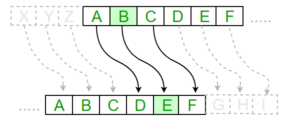# Substitution Cipher

• Difficulty Level : Medium
• Last Updated : 29 Sep, 2021

Hiding some data is known as encryption. When plain text is encrypted it becomes unreadable and is known as ciphertext. In a Substitution cipher, any character of plain text from the given fixed set of characters is substituted by some other character from the same set depending on a key. For example with a shift of 1, A would be replaced by B, B would become C, and so on.

Note: Special case of Substitution cipher is known as Caesar cipher where the key is taken as 3.

#### Mathematical representation

The encryption can be represented using modular arithmetic by first transforming the letters into numbers, according to the scheme, A = 0, B = 1,…, Z = 25. Encryption of a letter by a shift n can be described mathematically as.(Encryption Phase with shift n)(Decryption Phase with shift n)Examples:

```Plain Text: I am studying Data Encryption
Key: 4
Output: M eq wxyhCmrk Hexe IrgvCtxmsr

Plain Text: ABCDEFGHIJKLMNOPQRSTUVWXYZ
Key: 4
Output: EFGHIJKLMNOPQRSTUVWXYZabcd```

Algorithm for Substitution Cipher:

Input:

• A String of both lower and upper case letters, called PlainText.
• An Integer denoting the required key.

Procedure:

• Create a list of all the characters.
• Create a dictionary to store the substitution for all characters.
• For each character, transform the given character as per the rule, depending on whether we’re encrypting or decrypting the text.
• Print the new string generated.

Below is the implementation.

## Python3

 `# Python program to demonstrate` `# Substitution Cipher`     `import` `string`     `# A list containing all characters` `all_letters``=` `string.ascii_letters` ` `  `    `  `""" ` `create a dictionary to store the substitution ` `for the given alphabet in the plain text ` `based on the key` `"""` ` `  `    `  `dict1 ``=` `{}` `key ``=` `4` ` `  `for` `i ``in` `range``(``len``(all_letters)):` `    ``dict1[all_letters[i]] ``=` `all_letters[(i``+``key)``%``len``(all_letters)]` ` `  ` `  `plain_txt``=` `"I am studying Data Encryption"` `cipher_txt``=``[]` ` `  `# loop to generate ciphertext` ` `  `for` `char ``in` `plain_txt:` `    ``if` `char ``in` `all_letters:` `        ``temp ``=` `dict1[char]` `        ``cipher_txt.append(temp)` `    ``else``:` `        ``temp ``=``char` `        ``cipher_txt.append(temp)` `        `  `cipher_txt``=` `"".join(cipher_txt)` `print``(``"Cipher Text is: "``,cipher_txt)` ` `  `    `  `""" ` `create a dictionary to store the substitution` `for the given alphabet in the cipher ` `text based on the key` `"""` ` `  `    `  `dict2 ``=` `{}     ` `for` `i ``in` `range``(``len``(all_letters)):` `    ``dict2[all_letters[i]] ``=` `all_letters[(i``-``key)``%``(``len``(all_letters))]` `     `  `# loop to recover plain text` `decrypt_txt ``=` `[]`   `for` `char ``in` `cipher_txt:` `    ``if` `char ``in` `all_letters:` `        ``temp ``=` `dict2[char]` `        ``decrypt_txt.append(temp)` `    ``else``:` `        ``temp ``=` `char` `        ``decrypt_txt.append(temp)` `        `  `decrypt_txt ``=` `"".join(decrypt_txt)` `print``(``"Recovered plain text :"``, decrypt_txt) `

Output:

```Cipher Text is:  M eq wxyhCmrk Hexe IrgvCtxmsr
Recovered plain text : I am studying Data Encryption```

My Personal Notes arrow_drop_up
Recommended Articles
Page :